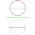## 2011/06/01

### The Background

The image I used as the background, at least the one used when I created this post, is a Voronoi diagram. To draw this diagram, we don't use any algorithm to find the vertices and edges of the diagram.

Our procedure consists first of splitting the unit square in YxY cells where each cells contains between 1 and X points. To render the image, that is a square of ZxZ pixels, we first convert the coordinate of the pixel into the unit square, then find in which cell the pixel is.

To draw the diagram, we need the two closest points from that pixel. To find it we first compare the pixel with other points in its cell. Then we also explore the surrounding cells to be sure to find the exact two points. When a cell is on the border of the square we use the cell on the other side to create a texture that can be tiled.

Let d1 and d2 be the distance from the pixel to the closest and second closest point respectively. Then, we can determined the gray color of that pixel as (d1/d2) * 255. Other method can be used too to determined the right color. The ratio d1/d2 gets a value of 0 when the pixel is right on the closest point and a value of 1 when the pixel is right between the two points. Therefore, in this case, the white pixels are part of a segment on the Voronoi diagram. The following image was created with this approach.

For our image in the background, we use an interpolation to determined the color for the pixel. Plus, we used d3, the distance from the third closest point to the pixel.

For the interpolation, we solve the following linear system:

| 0 0 1 || x |    | 1 |
| 1 1 1 || y | = | 0 |
| a b 0 || z |    | 0 |

where a and b are two different constants.

Then, the color of the pixel is determined by 255 * ((d1/d2) * x + (d1/d3) * y + z). Naturally, if the value is above 255 or below 0, we considered them as 255 or 0.

To program this procedure, we used CUDA. With CUDA, each pixel gets his own thread and even with an older version of CUDA (I just have CUDA 1.1 on my GeForce 9800 GTX+) the computation is done in less than a second for a 1024x1024 images.

The work load associated with a pixel is determined by the value X, the maximum number of point in a cell. To find the two (or three) closest point to a pixel, the maximum number of point to check is 9X. The memory required for the diagram is proportional to Y*Y*X.

REFERENCE

Steven Worley. 1996. A cellular texture basis function. In Proceedings of the 23rd annual conference on Computer graphics and interactive techniques (SIGGRAPH '96). ACM, New York, NY, USA, 291-294. DOI=10.1145/237170.237267 http://doi.acm.org/10.1145/237170.237267

1.I didn't know that's how CUDA worked. Very informative. Too bad my old GeForce 8800 probably doesn't have CUDA.

2.Actually, based on that web page http://developer.nvidia.com/cuda-gpus your GeForce 8800 can run CUDA 1.1 just like me.

All you need to do is go on the web site and download the driver for your card. Then, download the SDK and compile some of the examples. I strongly suggest to compile first "deviceQuery" which will show you exactly what you have on your card.

Then, to compare the speed for CUDA vs your CPU(s), I recommend Mandelbrot. By pressing 's', you can switch between the CPU and GPU implementation of the fractal. On my computer, for the CPU it's around 3 fps and for the GPU, 70 fps. So the difference is enormous.

Give me some feedbacks if you try it!